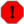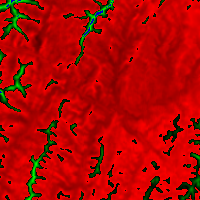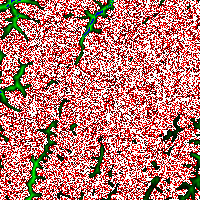## Name

ST_MapAlgebraExpr — 1 raster band version: Creates a new one band raster formed by applying a valid PostgreSQL algebraic operation on the input raster band and of pixeltype provided. Band 1 is assumed if no band is specified.

## Synopsis

`raster ST_MapAlgebraExpr(`raster rast, integer band, text pixeltype, text expression, double precision nodataval=NULL`)`;

`raster ST_MapAlgebraExpr(`raster rast, text pixeltype, text expression, double precision nodataval=NULL`)`;

## DescriptionST_MapAlgebraExpr is deprecated as of 2.1.0. Use ST_MapAlgebra instead.

Creates a new one band raster formed by applying a valid PostgreSQL algebraic operation defined by the `expression` on the input raster (`rast`). If no `band` is specified band 1 is assumed. The new raster will have the same georeference, width, and height as the original raster but will only have one band.

If `pixeltype` is passed in, then the new raster will have a band of that pixeltype. If pixeltype is passed NULL, then the new raster band will have the same pixeltype as the input `rast` band.

In the expression you can use the term `[rast]` to refer to the pixel value of the original band, `[rast.x]` to refer to the 1-based pixel column index, `[rast.y]` to refer to the 1-based pixel row index.

Availability: 2.0.0

## Examples

Create a new 1 band raster from our original that is a function of modulo 2 of the original raster band.

```ALTER TABLE dummy_rast ADD COLUMN map_rast raster;
UPDATE dummy_rast SET map_rast = ST_MapAlgebraExpr(rast,NULL,'mod([rast],2)') WHERE rid = 2;

SELECT
ST_Value(rast,1,i,j) As origval,
ST_Value(map_rast, 1, i, j) As mapval
FROM dummy_rast
CROSS JOIN generate_series(1, 3) AS i
CROSS JOIN generate_series(1,3) AS j
WHERE rid = 2;

origval | mapval
---------+--------
253 |      1
254 |      0
253 |      1
253 |      1
254 |      0
254 |      0
250 |      0
254 |      0
254 |      0
```

Create a new 1 band raster of pixel-type 2BUI from our original that is reclassified and set the nodata value to be 0.

```ALTER TABLE dummy_rast ADD COLUMN map_rast2 raster;
UPDATE dummy_rast SET
map_rast2 = ST_MapAlgebraExpr(rast,'2BUI','CASE WHEN [rast] BETWEEN 100 and 250 THEN 1 WHEN [rast] = 252 THEN 2 WHEN [rast] BETWEEN 253 and 254 THEN 3 ELSE 0 END', '0')
WHERE rid = 2;

SELECT DISTINCT
ST_Value(rast,1,i,j) As origval,
ST_Value(map_rast2, 1, i, j) As mapval
FROM dummy_rast
CROSS JOIN generate_series(1, 5) AS i
CROSS JOIN generate_series(1,5) AS j
WHERE rid = 2;

origval | mapval
---------+--------
249 |      1
250 |      1
251 |
252 |      2
253 |      3
254 |      3

SELECT
ST_BandPixelType(map_rast2) As b1pixtyp
FROM dummy_rast
WHERE rid = 2;

b1pixtyp
----------
2BUI
```original (column rast-view)rast_view_ma

Create a new 3 band raster same pixel type from our original 3 band raster with first band altered by map algebra and remaining 2 bands unaltered.

```SELECT